Surface Area of a Cylinder

In real life there are many examples of cylinders like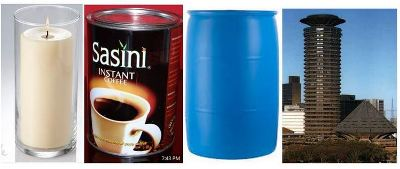All cylinders have a base, a top and a curved face.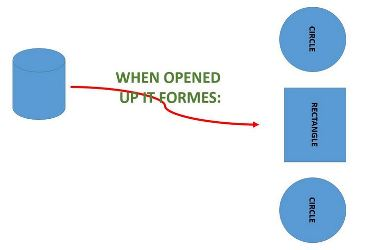Learn how to calculate the surface area of a cylinder in this video.

From the images and video viewed earlier:

Surface Area of a cylinder is the Area of top and bottom plus Area of the curved side.

Therefore,

Surface Area = 2(Area of circular top) + (circumference of top) by height of cylinder

#### Surface Area = 2(Area of a circle) + (Area of a rectangle)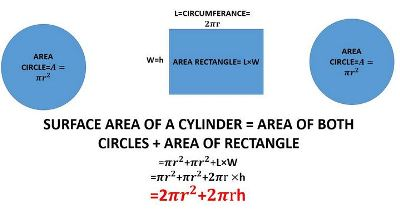#### Questions

Attempt to answer the questions before studying the solution given.

Question 1.

A cylindrical can 12cm high has a radius of 8.8cm. What is the surface area of the curved part?( use pie as 3.14)

#### Solution to Question 1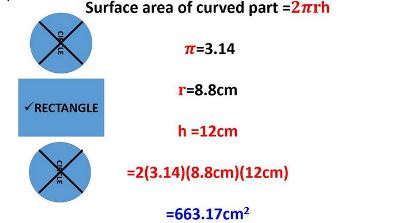Question 2.

An open cylinder has a diameter of 12.8cm and a height of 20cm. Find the surface area of the cylinder.

(Use pie as 3.14)

#### Solution to Question 2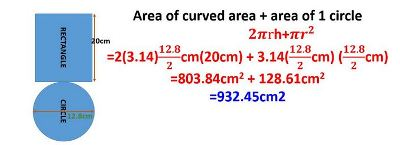#### Question 3

Kajairo build a cylindrical tank at his home measuring 6m high and 2.6m in diameter. Find the surface area of the tank.

#### Solution to Question 3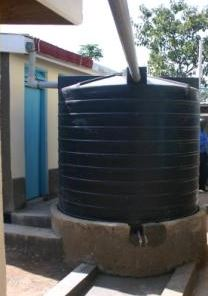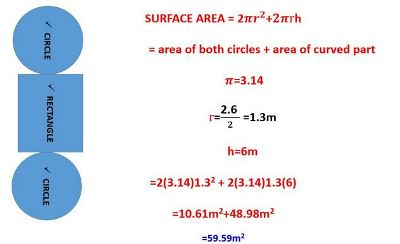For more exercises, look at your text book.